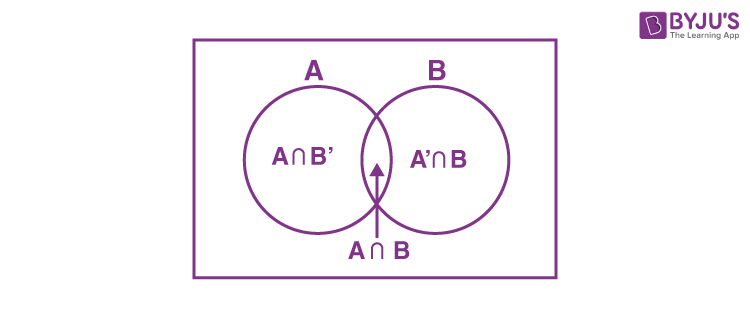# Independent Events And Probability

Independent events are those events whose occurrence is not dependent on any other event. For example, if we flip a coin in the air and get the outcome as Head, then again if we flip the coin but this time we get the outcome as Tail. In both cases, the occurrence of both events is independent of each other. It is one of the types of events in probability. Let us learn here the complete definition of independent events along with its Venn diagram, examples and how it is different from mutually exclusive events.

## What are Independent Events?

In Probability, the set of outcomes of an experiment is called events. There are different types of events such as independent events, dependent events, mutually exclusive events, and so on.

If the probability of occurrence of an event A is not affected by the occurrence of another event B, then A and B are said to be independent events.

Consider an example of rolling a die. If A is the event ‘the number appearing is odd’ and B be the event ‘the number appearing is a multiple of 3’, then

P(A)= 3/6 = 1/2 and P(B) = 2/6 = 1/3

Also A and B is the event ‘the number appearing is odd and a multiple of 3’ so that

P(A ∩ B) = 1/6

P(A│B) = P(A ∩ B)/ P(B)

$$\begin{array}{l}=\frac{\frac{1}{6}}{\frac{1}{3}}=\frac{1}{2}\end{array}$$

P(A) = P(A│B) = 1/2  , which implies that the occurrence of event B has not affected the probability of occurrence of the event A .

If A and B are independent events, then P(A│B) = P(A)

Using the Multiplication rule of probability, P(A ∩ B) = P(B) .P(A│B)

P(A ∩ B) = P(B) .P(A)Note: A and B are two events associated with the same random experiment, then A and B are known as independent events if P(A ∩ B) = P(B) .P(A)

What are Mutually Exclusive Events?

Two events A and B are said to be mutually exclusive events if they cannot occur at the same time. Mutually exclusive events never have an outcome in common.

### Independent Events Vs Mutually Exclusive Events

The difference between the independent events and mutually exclusive events are given below:

 Independent Events Mutually exclusive events They cannot be specified based on the outcome of a maiden trial. They are independent of trials Can have common outcomes Can never have common outcomes If A and B are two independent events, then P(A ∩ B) = P(B) .P(A) If A and B are two mutually exclusive events, then P(A ∩ B) = 0

## Independent Events Venn Diagram

Let us proof the condition of independent events using a Venn diagram.

Theorem: If X and Y are independent events, then the events X and Y’ are also independent.

Proof: The events A and B are independent, so, P(X ∩ Y) = P(X) P(Y).

Let us draw a Venn diagram for this condition:From the Venn diagram, we see that the events X ∩ Y and X ∩ Y’ are mutually exclusive, and together they form the event X.

X = ( X ∩ Y) ∪ (X ∩ Y’)
Also, P(X) = P[(X ∩ Y) ∪ (X ∩ Y’)] or, P(X) = P(X ∩ Y) + P(X ∩ Y’)
or, P(X) = P(X) P(Y) + P(X ∩ Y’)
or, P(X inter Y’) = P(X) − P(X) P(Y) = P(X) (1 – P(Y)) = P(X) P(Y’)

## Example with Solution

Question: Let X and Y are two independent events such that P(X) = 0.3 and P(Y) = 0.7. Find P(X and Y), P(X or Y), P(Y not X), and P(neither X nor Y).

Solution: Given P(X) = 0.3 and P(Y) = 0.7 and events X and Y are independent of each other.

P(X and Y) = P( X ∩ Y) = P(X) P(Y) = 0.3 × 0.7 = 0.21

P(X or Y) = P(X ∪ Y) = P(X) + P(Y) – P(X ∩ Y) = 0.3 + 0.7 – 0.21 = 0.79

P(Y not X) = P(Y ∩ X’) = P(Y) – P(X ∩ Y) = 0.7 – 0.21 = 0.49

And P(neither X nor Y) = P(X’ ∩ Y’) = 1 – P(X ∪ Y) = 1 – 0.79 = 0.21

To know more about independent events in probability, download our BYJU’S – The Learning App and convert learning into an interesting hobby.

Test your knowledge on independent events